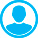SHAPE DIFFERENTIABILITY OF DRAG FUNCTIONAL AND BOUNDARY VALUE PROBLEM SOLUTIONS FOR FLUID MIXTURE EQUATIONSAbstract (English):
Problems of optimal design of various elements of technical structures stimulate mathematical statements of new problems of continuum mechanics and hydrodynamics in particular. This study refers to problems of shape optimization of profiles in a flow of fluid or gas. The paper deals with properties of solutions and their functional of inhomogeneous boundary value problem for nonlinear composite type partial differential equation system, simulating the a mixture of viscous compressible fluids (gases) flowing around an obstacle. Methods of the theory of partial differential equations, functional analysis and, in particular, the results on the solvability of boundary value problems for transport and Stokes equations established the well-posedness of a linear boundary value problem with singular coefficients (the problem of the original problem solution difference). This result allowed to obtain the uniqueness theorem to determine the nature of solutions dependence on the shape of the flow range and to prove domain differentiability of the solutions considered. Domain differentiability of the solution functional reflecting the force of the obstacle resistance to the incident flow is proved. A formula to equate this derivative as a sum of two summands, one of which clearly depends on mapping setting the domain shape, and the other can be expressed in terms of the so-called adjoint state, depending only on the solution of the original problem in a non-deformed domain. The functional derivative formulas may be used as the basis for building a numerical algorithm for finding the optimal shape of the body in a flow of mixture of viscous compressible fluids.

Keywords:
boundary value problem, mixture of viscous compressible fluids, conjugate problem, flowing around an obstacle, material derivative, shape derivative

1. Novo S. Compressible Navier-Stokes model with inflow-outflow boundary conditions. J. Math. Fluid Mech., 2005, vol. 7, pp. 485-514.

2. Girinon V. Navier-Stokes equations with nonhomogeneous boundary conditions in a bounded three-dimensional domain. J. Math. Fluid Mech., 2011, vol. 13, pp. 309-339.

3. Farwig R. Stationary solutions of compressible Navier-Stokes equations with slip boundary condition. Comm. Partial Dierential Equations, 1989, vol. 14, pp. 1579-1606.

4. Kweon J. R., Kellogg R. B. Compressible Navier-Stokes equations in a bounded domain with inflow boundary condition. SIAM J. Math. Anal., 1997, vol. 28, pp. 94-108.

5. Kweon J. R., Kellogg R. B. Regularity of solutions to the Navier-Stokes equations for compressible barotropic flows on a polygon.

6. Arch. Ration. Mech. Anal. 2000, vol. 163, pp. 35-64.

7. Plotnikov P.I., Sokolovski Zh. Statsionarnye kraevye zadachi dlya uravneniy Nav'e-Stoksa s pokazatelem adiabaty [Boundary Value Problems for Navier-Stokes Equations with Adiabatic Exponent y № 3/2 ]. Doklady Akademii Nauk [Proc. of the RAS], 2004, vol. 397, no. 2, pp. 166-169.

8. Plotnikov P., Sokolowski J.Compressible Navier-Stokes equations: Theory and shape optimization. Basel: Springer, 2012. 474 p.

9. Plotnikov P. I., Ruban E. V., Sokolowski J. Inhomogeneous boundary value problems for compressible Navier-Stokes and transport equations.J. Math. Pures Appl., 2009, vol. 92, pp. 113-162.

10. Zhalnina A.A., Kucher N.A. On the Well-Posedness of an Inhomogeneous Boundary Value Problem for the Equations of Mixtures of Viscous Compressible Fluids. Journal of Applied and Industrial Mathematics, 2015, vol. 9, no. 4, pp. 598-610. doi: 10.1134/ S19904789I504016X

11. Sokolowski J., Zolesio J.-P. Intro duction to Shape Optimization. Shape Sensitivity Analysis. Springer Ser. Comput. Math. 16.

12. Berlin: Springer, 1992. 250 p.

13. Bucur D., Buttazzo G.Variational Methods in Shape Optimization Problems. Boston: Birkhauser, 2005. 216 p.

14. Delfour M.C., Zolesio J.-P.Shapes and Geometries, Adv. Design Control 22. Philadelphia: SIAM, 2011. 501 p.

15. Henrot A., Pierre M.Variation et optimisation de formes: une analyse geometrique. Paris: Springer, 2005. 334 p.

16. Kawohl B., Pironneau 0., Tartar L., Zolesio J.-P.Optimal Shape Design, Lecture Notes in Math.Berlin: Springer, 2000. 388 p.

17. Novotny A., Sokolowski J. Topological Derivatives in Shape Optimization. Series: Interaction of Mechanics and Mathematics.

18. Berlin: Springer, 2013. 412 p.

19. Feireisl E. Shape optimization in viscous compressible fluids. Appl. Math. Optim., 2003, vol. 47, pp. 59-78.

20. Plotnikov P., Sokolowski J. Shape derivative of drag functional.SIAM J.Control Optim., 2010, vol. 48, pp. 4680--4706.

21. Plotnikov P.I., Ruban E.V., Sokolowski J. Inhomogeneous boundary value problems for compressible Navier-Stokes equations, well-posedness and sensitivity analysis.SIAM J. Math. Anal., 2008, vol. 40, pp. 1152-1200

22. Plotnikov P., Sokolowski J. Domain dependence of solutions to compressible Navier-Stokes equations. SIAM J. Control Optim,. 2006, vol. 45, pp. 1165-1197.

23. Plotnikov P., Sokolowski J. On compactness, domain dependence and existence of steady state solutions to compressible isothermal Navier-Stokes equations.J. Math. Fluid Mech., 2005, vol. 7, pp. 529-573.

24. Rajagopal K. R., Tao L.Mechanics of mixtures. Singapore: World Sci., 1995. 195 p.

25. Krayko A.N., Nigmatulin R.I. Mekhanika mnogofaznykh sred [Mechanics of multiphase media]. Itogi nauki i tehniki. Seriya: gidromekhanika [Results of science and technology. Series: Hydromechanics], 1972, vol. 6, pp. 93-174.

26. Nigmatulin R.I.Dinamika mnogofaznykh sred. Chast' l [Dynamics of multiphase media] Part 1. Moscow: Nauka Puhl., 1987. 464 p.

27. Sobolev S. L.Nekotorye primeneniya funktsional'nogo analiza v matematicheskoy fizike [Some applications of functional analysis in mathematical physics]. Moscow: Nauka Puhl., 1988. 333 p.

28. 25 Berg Y., Lefstrem Y. Interpolyatsionnye prostranstva. Vvedenie [Interpolation spaces. Introduction]. Moscow: Mir Puhl., 1980. 264 p.

29. 26. Galdi G. An introduction to the mathematical theory of the Navier-Stokes equations: Steady-State Problems. Springer Monographs in Mathematics. New-York: Springer Science, 2011. 1018 p.